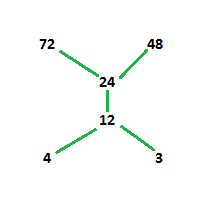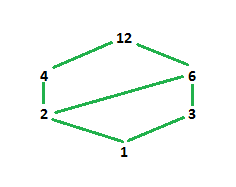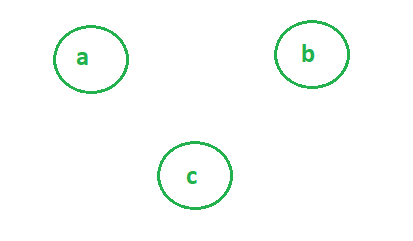GeeksforGeeks App
Open AppBrowser
Continue

# Discrete Mathematics | Hasse Diagrams

A Hasse diagram is a graphical representation of the relation of elements of a partially ordered set (poset) with an implied upward orientation. A point is drawn for each element of the partially ordered set (poset) and joined with the line segment according to the following rules:

• If p<q in the poset, then the point corresponding to p appears lower in the drawing than the point corresponding to q.
• The two points p and q will be joined by line segment if p is related to q.

To draw a Hasse diagram, provided set must be a poset.
A poset or partially ordered set A is a pair, ( B,) of a set B whose elements are called the vertices of A and obeys following rules:

1. Reflexivity → pB
2. Anti-symmetric → pq and qp if p=q
3. Transitivity → if pq and qr then pr

Example-1: Draw Hasse diagram for ({3, 4, 12, 24, 48, 72}, /)

Explanation – According to above given question first, we have to find the poset for the divisibility.
Let the set is A.
A={(312), (324), (348), (372), (412), (424), (448), (472), (1224), (1248), (1272), (2448), (2472)}
So, now the Hasse diagram will be:In above diagram, 3 and 4 are at same level because they are not related to each other and they are smaller than other elements in the set. The next succeeding element for 3 and 4 is 12 i.e, 12 is divisible by both 3 and 4. Then 24 is divisible by 3, 4 and 12. Hence, it is placed above 12. 24 divides both 48 and 72 but 48 does not divide 72. Hence 48 and 72 are not joined.
We can see transitivity in our diagram as the level is increasing.

Example-2: Draw Hasse diagram for (D, /)

Explanation – Here, Dmeans set of positive integers divisors of 12.
So, D={1, 2, 3, 4, 6, 12}
poset A = {(12), (13), (14), (16), (112), (24), (26), (212), (36), (312), (412), (612)}
So, now the Hasse diagram will be-In above diagram, 1 is the only element that divides all other elements and smallest. Hence, it is placed at the bottom. Then the elements in our set are 2 and 3 which do not divide each other hence they are placed at same level separately but divisible by 1 (both joined by 1). 4 is divisible by 1 and 2 while 6 is divisible by 1, 2 and 3 hence, 4 is joined by 2 and 6 is joined by 2 and 3. 12 is divisible by all the elements hence, joined by 4 and 6 not by all elements because we have already joined 4 and 6 with smaller elements accordingly.

For regular Hasse Diagram:

• Maximal element is an element of a POSET which is not less than any other element of the POSET. Or we can say that it is an element which is not related to any other element. Top elements of the Hasse Diagram.
• Example- In the diagram above, we can say that 4,2,3,6,1 are related to 12 (ordered by division e.g. (4,/) ) but 12 is not related to any other. (As Hasse Diagram is upward directional).
• Minimal element is an element of a POSET which is not greater than any other element of the POSET. Or we can say that no other element is related to this element. Bottom elements of the Hasse Diagram.
• Example- In the diagram above, we can say that 1 is related to 2,3,4,6,12 (ordered by division e.g. (4,/) ) but no element is related to 1. (As Hasse Diagram is upward directional).
• Greatest element (if it exists) is the element succeeding all other elements.
• Least element is the element that precedes all other elements.

Note – Greatest and Least element in Hasse diagram are only one.

In Example-1,
Maximal elements are 48 and 72 since they are succeeding all the elements.
Minimal elements are 3 and 4 since they are preceding all the elements.
Greatest element does not exist since there is no any one element that succeeds all the elements.
Least element does not exist since there is no any one element that precedes all the elements.

In Example-2,
Maximal and Greatest element is 12 and Minimal and Least element is 1.

NOTE: An element can be both maximal and minimal

Example-Here a,b,c are maximal as well as minimal.

My Personal Notes arrow_drop_up